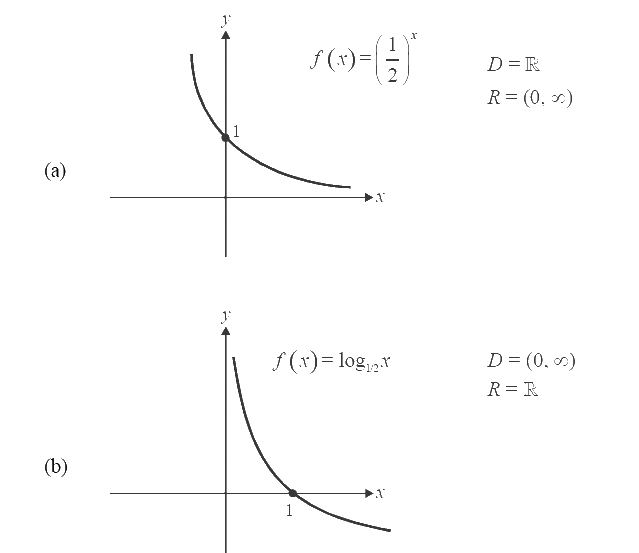# Examples on Exponential and Logarithmic Functions Set 1

Go back to  'Functions'

Example- 25

Find the domain and range of these functions and plot their graphs:

(a) \begin{align}f(x) = {\left( {\frac{1}{2}} \right)^x}\end{align}                (b) $$f(x) = {\log _{1/2}}x$$

Solution: We are just providing the answers. The reasoning is left to the student as an exercise.Example- 26

Find the domains of the following functions.

(a) $$f(x) = \log |{x^2} - 3x + 2|$$              (b) $$f(x) = \log ([x])$$

Solution:

(a) For the log function to be defined, we require the argument to be positive. For the given function, the argument is $$\left| {{x^{2{\rm{ }}}} - 3x + {\rm{ }}2} \right|$$, which is everywhere positive except the points where it can be 0; we need to exclude these points from the domain.

${x^2} - 3x + 2 = 0{\rm{ }}\ \quad \Rightarrow \quad (x - 1)(x - 2) = 0 \quad \Rightarrow \quad x = 1,2\\ D = {\rm{\mathbb{R}}} - \left\{ {1,2} \right\}$

(b) We require the argument to the log function to be positive.

$[x] > 0{\rm{ }} \quad \Rightarrow \quad {\text{ This is possible only when }}x > 1\\D = [1,{\rm{ }}\infty )$

Example- 27

Find the domain of $$f(x) = {\log _2}\;{\log _3}\;{\log _4}\;{\log _5}\;x$$

Solution: By definition, logarithmic function is defined only when its argument is positive.

Here we have four ‘logs’. We start with the outermost log’s argument. This argument should be positive

$$\Rightarrow {\log_3}{\log_4}{\log_5}x > 0$$

By observation (or by taking successive antilogs in the order base 3, base 4, base 5), we get

$$\Rightarrow {\log_4}{\log_5}x > {3^0} = 1$$

$$\Rightarrow {\log_5}x > {4^1} = 4$$

$$\Rightarrow x > {5^4} = 625$$

Therefore,       $$D = (625,{\rm{ }}\infty )$$